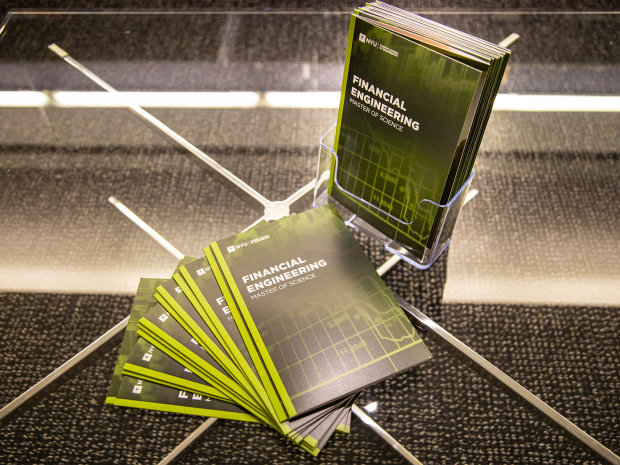B.S./M.S. Program in Financial Engineering | NYU Tandon School of Engineering

# B.S./M.S. Program in Financial EngineeringThe Department of Finance and Risk Engineering offers an accelerated B.S./M.S. Program to undergraduates with strong academic records and an interest in Financial Engineering, which will lead to the award of both a Bachelor's and a Master's degree from NYU Tandon in five years.

The prerequisites for this program are:

4 Credits Introduction to Programming & Problem Solving CS-UY1114
This course introduces problem solving and computer programming and is for undergraduate Computer Science and Computer Engineering majors who have limited prior experience in programming in any language. The course covers fundamentals of computer programming and its underlying principles using the Python programming language. Concepts and methods introduced in the course are illustrated by examples from various disciplines. ABET competencies: a,b,c, e, f, g, k
Corequisite: EX-UY 1; Anti-requisite: CS-UY 1113

4 Credits Linear Algebra and Differential Equations MA-UY2034
MA-UY 2034 is an introduction to ordinary differential equations and linear algebra. The course develops the techniques for the analytic and numeric solutions of ordinary differential equations (and systems) that are widely used in modern engineering and science. Linear algebra is used as a tool for solving systems of linear equations as well as for understanding the structure of solutions to linear (systems) of differential equations. Topics covered include the fundamental concepts of linear algebra such as Gaussian elimination, matrix theory, linear transformations, vector spaces, subspaces, basis, eigenvectors, eigenvalues and the diagonalization of matrices, as well as the techniques for the analytic and numeric solutions of ordinary differential equations (and systems) that commonly appear in modern engineering and science.
Prerequisite: MA-UY 1124, MA-UY 1424 or MA-UY 1132. Note: Not open to students who have taken MA-UY 3044 or MA-UY 3054 or MA-UY 3083 or MA-UY 4204.

4 Credits Data Analysis MA-UY2224
An introductory course to probability and statistics. It affords the student some acquaintance with both probability and statistics in a single term. Topics in Probability include mathematical treatment of chance; combinatorics; binomial, Poisson, and Gaussian distributions; the Central Limit Theorem and the normal approximation. Topics in Statistics include sampling distributions of sample mean and sample variance; normal, t-, and Chi-square distributions; confidence intervals; testing of hypotheses; least squares regression model. Applications to scientific, industrial, and financial data are integrated into the course.NOTE: Not open to students who have taken MA-UY 2233 or MA-UY 3012 or MA-UY 3022.
Prerequisite: MA-UY 1124, MA-UY1424, or MA-UY 1132 or MATH-UH 1020 or MATH-UH 1021 or MATH-SHU 151

The corequisite for this program is:

4 Credits Calculus III: Multi-dimensional Calculus MA-UY2114
Vectors in the plane and space. Partial derivatives with applications, especially Lagrange multipliers. Double and triple integrals. Spherical and cylindrical coordinates. Surface and line integrals. Divergence, gradient, and curl. Theorems of Gauss and Stokes.
Prerequisite: MA-UY 1124 or MA-UY 1424 or MA-UY 1132. Anti-requisite: MA-UY 2514

Qualified students are typically considered for admission to the program during their junior year. In their remaining undergraduate semesters, they will complete some graduate coursework during regular terms and/or during the summer towards their desired master's degree.